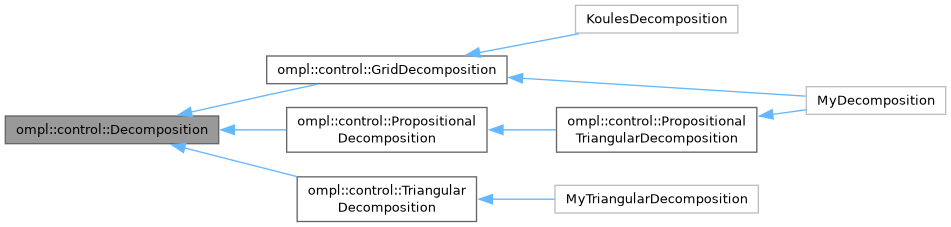ompl::control::Decomposition Class Referenceabstract

A Decomposition is a partition of a bounded Euclidean space into a fixed number of regions which are denoted by integers. More...

#include <ompl/control/planners/syclop/Decomposition.h>

Inheritance diagram for ompl::control::Decomposition:[legend]

## Public Member Functions

Decomposition (int dim, const base::RealVectorBounds &b)
Constructor. Creates a Decomposition with a given dimension and a given set of bounds. Accepts as an optional argument a given number of regions.

virtual int getNumRegions () const =0
Returns the number of regions in this Decomposition.

virtual int getDimension () const
Returns the dimension of this Decomposition.

virtual const base::RealVectorBoundsgetBounds () const
Returns the bounds of this Decomposition.

virtual double getRegionVolume (int rid)=0
Returns the volume of a given region in this Decomposition.

virtual int locateRegion (const base::State *s) const =0
Returns the index of the region containing a given State. Most often, this is obtained by first calling project(). Returns -1 if no region contains the State.

virtual void project (const base::State *s, std::vector< double > &coord) const =0
Project a given State to a set of coordinates in R^k, where k is the dimension of this Decomposition.

virtual void getNeighbors (int rid, std::vector< int > &neighbors) const =0
Stores a given region's neighbors into a given vector.

virtual void sampleFromRegion (int rid, RNG &rng, std::vector< double > &coord) const =0
Samples a projected coordinate from a given region.

virtual void sampleFullState (const base::StateSamplerPtr &sampler, const std::vector< double > &coord, base::State *s) const =0
Samples a State using a projected coordinate and a StateSampler.

## Protected Attributes

int dimension_

base::RealVectorBounds bounds_

## Detailed Description

A Decomposition is a partition of a bounded Euclidean space into a fixed number of regions which are denoted by integers.

Definition at line 126 of file Decomposition.h.

The documentation for this class was generated from the following file: